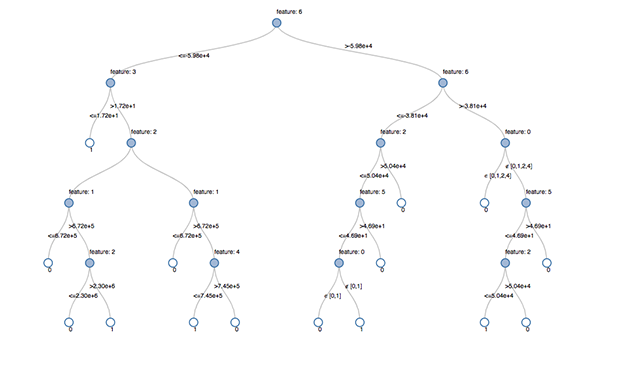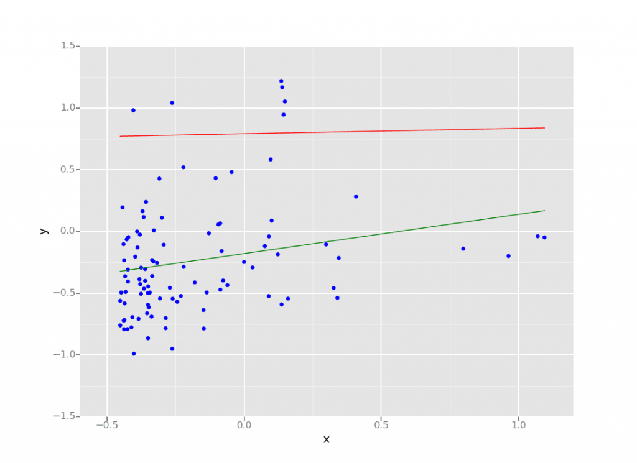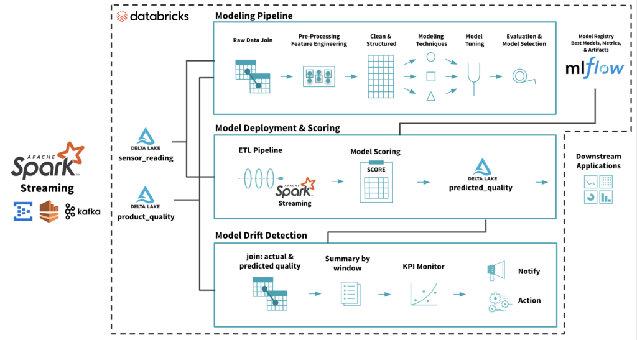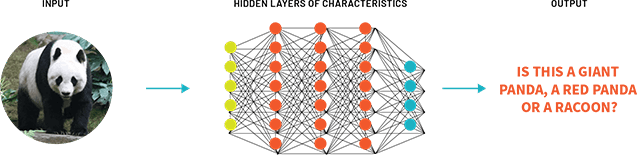## What is a machine learning Model?

A machine learning model is a program that can find patterns or make decisions from a previously unseen dataset. For example, in natural language processing, machine learning models can parse and correctly recognize the intent behind previously unheard sentences or combinations of words. In image recognition, a machine learning model can be taught to recognize objects - such as cars or dogs. A machine learning model can perform such tasks by having it 'trained' with a large dataset. During training, the machine learning algorithm is optimized to find certain patterns or outputs from the dataset, depending on the task. The output of this process - often a computer program with specific rules and data structures - is called a machine learning model.

## What is a machine learning Algorithm?

A machine learning algorithm is a mathematical method to find patterns in a set of data. Machine Learning algorithms are often drawn from statistics, calculus, and linear algebra. Some popular examples of machine learning algorithms include linear regression, decision trees, random forest, and XGBoost.

## What is Model Training in machine learning?

The process of running a machine learning algorithm on a dataset (called training data) and optimizing the algorithm to find certain patterns or outputs is called model training. The resulting function with rules and data structures is called the trained machine learning model.

## What are the different types of Machine Learning?

In general, most machine learning techniques can be classified into supervised learning, unsupervised learning, and reinforcement learning.

## What is Supervised Machine Learning?

In supervised machine learning, the algorithm is provided an input dataset, and is rewarded or optimized to meet a set of specific outputs. For example, supervised machine learning is widely deployed in image recognition, utilizing a technique called classification. Supervised machine learning is also used in predicting demographics such as population growth or health metrics, utilizing a technique called regression.

## What is Unsupervised Machine Learning?

In unsupervised machine learning, the algorithm is provided an input dataset, but not rewarded or optimized to specific outputs, and instead trained to group objects by common characteristics. For example, recommendation engines on online stores rely on unsupervised machine learning, specifically a technique called clustering.

## What is Reinforcement Learning?

In reinforcement learning, the algorithm is made to train itself using many trial and error experiments. Reinforcement learning happens when the algorithm interacts continually with the environment, rather than relying on training data. One of the most popular examples of reinforcement learning is autonomous driving.

## What are the different machine learning models?

There are many machine learning models, and almost all of them are based on certain machine learning algorithms. Popular classification and regression algorithms fall under supervised machine learning, and clustering algorithms are generally deployed in unsupervised machine learning scenarios.

#### Supervised Machine Learning

• Logistic Regression: Logistic Regression is used to determine if an input belongs to a certain group or not
• SVM: SVM, or Support Vector Machines create coordinates for each object in an n-dimensional space and uses a hyperplane to group objects by common features
• Naive Bayes: Naive Bayes is an algorithm that assumes independence among variables and uses probability to classify objects based on features
• Decision Trees: Decision trees are also classifiers that are used to determine what category an input falls into by traversing the leaf's and nodes of a tree
• Linear Regression: Linear regression is used to identify relationships between the variable of interest and the inputs, and predict its values based on the values of the input variables.
• kNN: The k Nearest Neighbors technique involves grouping the closest objects in a dataset and finding the most frequent or average characteristics among the objects.
• Random Forest: Random forest is a collection of many decision trees from random subsets of the data, resulting in a combination of trees that may be more accurate in prediction than a single decision tree.
• Boosting algorithms: Boosting algorithms, such as Gradient Boosting Machine, XGBoost, and LightGBM, use ensemble learning. They combine the predictions from multiple algorithms (such as decision trees) while taking into account the error from the previous algorithm.

#### Unsupervised Machine Learning

• K-Means: The K-Means algorithm finds similarities between objects and groups them into K different clusters.
• Hierarchical Clustering: Hierarchical clustering builds a tree of nested clusters without having to specify the number of clusters.

## What is a Decision Tree in Machine Learning (ML)?

A Decision Tree is a predictive approach in ML to determine what class an object belongs to. As the name suggests, a decision tree is a tree-like flow chart where the class of an object is determined step-by-step using certain known conditions.A decision tree visualized in the Databricks Lakehouse. Source: https://www.databricks.com/blog/2019/05/02/detecting-financial-fraud-at-scale-with-decision-trees-and-mlflow-on-databricks.html

## What is Regression in Machine Learning?

Regression in data science and machine learning is a statistical method that enables predicting outcomes based on a set of input variables. The outcome is often a variable that depends on a combination of the input variables.A linear regression model performed on the Databricks Lakehouse. Source: https://www.databricks.com/blog/2015/06/04/simplify-machine-learning-on-spark-with-databricks.html

## What is a Classifier in Machine Learning?

A classifier is a machine learning algorithm that assigns an object as a member of a category or group. For example, classifiers are used to detect if an email is spam, or if a transaction is fraudulent.

## How many models are there in machine learning?

Many! Machine learning is an evolving field and there are always more machine learning models being developed.

## What is the best model for machine learning?

The machine learning model most suited for a specific situation depends on the desired outcome. For example, to predict the number of vehicle purchases in a city from historical data, a supervised learning technique such as linear regression might be most useful. On the other hand, to identify if a potential customer in that city would purchase a vehicle, given their income and commuting history, a decision tree might work best.

## What is model deployment in Machine Learning (ML)?

Model deployment is the process of making a machine learning model available for use on a target environment—for testing or production. The model is usually integrated with other applications in the environment (such as databases and UI) through APIs. Deployment is the stage after which an organization can actually make a return on the heavy investment made in model development.A full machine learning model lifecycle on the Databricks Lakehouse. Source: https://www.databricks.com/blog/2019/09/18/productionizing-machine-learning-from-deployment-to-drift-detection.html

## What are Deep Learning Models?

Deep learning models are a class of ML models that imitate the way humans process information. The model consists of several layers of processing (hence the term 'deep') to extract high-level features from the data provided. Each processing layer passes on a more abstract representation of the data to the next layer, with the final layer providing a more human-like insight. Unlike traditional ML models which require data to be labeled, deep learning models can ingest large amounts of unstructured data. They are used to perform more human-like functions such as facial recognition and natural language processing.A simplified representation of deep learning. Source: https://www.databricks.com/discover/pages/the-democratization-of-artificial-intelligence-and-deep-learning

## What is Time Series Machine Learning?

A time-series machine learning model is one in which one of the independent variables is a successive length of time minutes, days, years etc.), and has a bearing on the dependent or predicted variable. Time series machine learning models are used to predict time-bound events, for example - the weather in a future week, expected number of customers in a future month, revenue guidance for a future year, and so on.